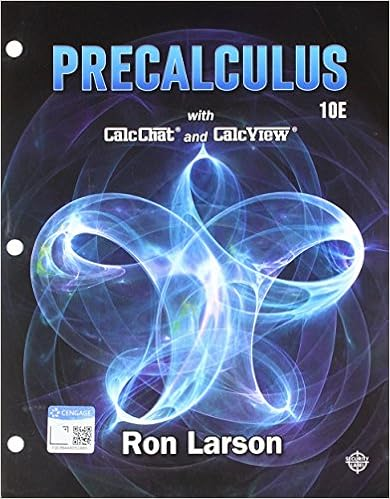# Write as an algebraic expression the quotient of a

• tutoringinaninstant
• 12

This preview shows page 11 - 12 out of 12 pages.

##### We have textbook solutions for you!
The document you are viewing contains questions related to this textbook.The document you are viewing contains questions related to this textbook.
Chapter 2 / Exercise 79
Precalculus
Hostetler/LarsonExpert Verified
29.Write as an algebraic expression:
30.Write as an algebraic expression:
##### We have textbook solutions for you!
The document you are viewing contains questions related to this textbook.The document you are viewing contains questions related to this textbook.
Chapter 2 / Exercise 79
Precalculus
Hostetler/LarsonExpert Verified
Module 2 - Fundamentals of Algebra | Page 27 Last Updated: 8/2/2017
31.Write the following phrase as a mathematical expression: Jason ran 3 times as far as Sean. Together they ran 36 miles. How far did each of them run?
32.Rita's score is 12 more than 2 times Milan's score. If we let zrepresent Milan's score, write an algebraic expression for Rita's score. 33.Linda and Juan went shopping. Linda spent \$11 less than Juan. If we let wrepresent how much Juan spent, write an algebraic expression for how much Linda spent.
34.One subtracted from ten times a number is 239. Find the number. 35.Five times the sum of nine and a number is -15. Find the number. 36.The sum of twice a number and 6 is 4. Find the number. 37.Four times the difference of a number and 1 is 8. Find the number.
38.A wire 20 cm long is cut into two pieces. The longer piece is 4 cm longer than the shorter piece. Find the length of the shorter piece of wire. 39.A rectangular field is two times as long as it is wide. If the perimeter of the field is 450 feet, what are the dimensions of the field? 40.Jason ran 3 times as far as Sean. Together they ran 36 miles. How far did each of them run?
•••